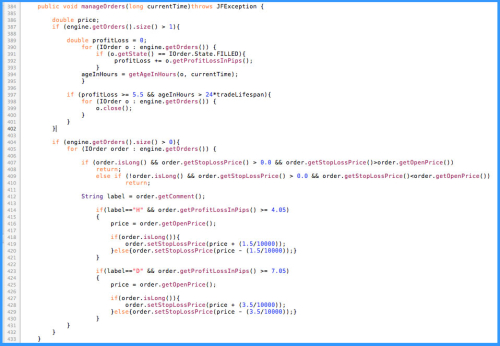In my last month article I described the general ideas of how I decided to automate the strategy presented by the member BlueDragon, as I mentioned I saw a great potential in it. In this article, the last of this year, I want to show you the practical part as regards the implementation of the code. Just picking up on what was described in the previous article, we will analyze step by step the generated code to build the strategy. I'm not going to show how a strategy is made from scratch, as it is already well explained in previous articles from other community members.

Let us start analyzing the code for each point described in the previous article:

1 - Pivots Calculation:
• I will start by presenting the main piece of code of the strategy, it is here that the pivots are calculated according to the periods used : H4 - Daily - Weekly and Monthly. What this piece of code does is get the history of the last candle of each period and then calculate the (H+L+C)/3. Then it checks the difference between the closing price of the candle and the pivot calculated. If the difference is greater than or equal to the defined, the conditions are set to open trades at the beginning of the next candle in the period in which this difference occurred.• Figure-1 - Code Sample for Pivots Calculation

2 - Orders Management:

After analyzed the behavior of the orders placed by the strategy, we decided to put a "Move to Break Even" in the orders opened by the H4 pivot and Daily pivot. So, what the next code example does is select the orders that were open for the time periods referred to, by selecting the comment "H" for H4 and "D" for Daily. Subsequently, if orders are found in the defined condition, the Stop Loss is placed at Break Even , which is also different for each case.• Figure-2 - Code Sample for Order Management

3 - Orders Reinforcement:

For orders that are opened in monthly and weekly pivots, as sometimes they take longer to close, we decided to make reinforcement with new orders, making a kind of "martingale". Thus, if the current price is further away from the pivot, the next piece of code analyzes the orders through the comments of each one, and if find open orders in those pivots with deviations from the opening price higher than the settings, opens an order of reinforcement. It should be noted that this increase is only done weekly for monthly orders and daily for weekly orders.• Figure-3 - Code Sample for Order Reinforcement

4 - Orders Placement:

Now that we've introduced the 3 main pieces of strategy code, let's see how they are invoked within the method onBAR(), to place orders within the defined periods. All orders are placed at market prices, so the code example below illustrates how the orders are sent to the platform, taking into account the Take Profit levels calculated for each order. As you can see in the code, the strategy reinforce orders on Daily and Weekly periods, for Weekly and Monthly orders respectively.• Figure-4 - Code Sample for Order Placement

Final Words:
Much more could be said about this strategy, but nothing better than seeing the results achieved so far. Here is an extract from the account where the strategy is running from October 17th until now, so you can get an idea of their performance. As the strategy is running remotely, it is noteworthy that it lost some trades due to upgrades made ​​on the platform, which led to the arrest of strategy. As I did not realize the time, the strategy was stopped for a few days.by: LM

Translate to English Show original